# Kendall's Tau-b using SPSS Statistics

## Introduction

Kendall's tau-b (τb) correlation coefficient (Kendall's tau-b, for short) is a nonparametric measure of the strength and direction of association that exists between two variables measured on at least an ordinal scale. It is considered a nonparametric alternative to the Pearson’s product-moment correlation when your data has failed one or more of the assumptions of this test. It is also considered an alternative to the nonparametric Spearman rank-order correlation coefficient (especially when you have a small sample size with many tied ranks). If you consider one of your variables as an independent variable and the other as a dependent variable, you might consider running a Somers' d test instead.

For example, you could use Kendall's tau-b to understand whether there is an association between exam grade and time spent revising (i.e., where there were six possible exam grades – A, B, C, D, E and F – and revision time was split into five categories: less than 5 hours, 5-9 hours, 10-14 hours, 15-19 hours, and 20 hours or more). Alternately, you could use Kendall's tau-b to understand whether there is an association between customer satisfaction and delivery time (i.e., where delivery time had four categories – next day, 2 working days, 3-5 working days, and more than 5 working days – and customer satisfaction was measured in terms of the level of agreement customers had with the following statement, "I am satisfied with the time it took for my parcel to be delivered", where the level of agreement had five categories: strongly agree, agree, neither agree nor disagree, disagree and strongly disagree).

This "quick start" guide shows you how to carry out Kendall's tau-b using SPSS Statistics. We show you the main procedure for doing this here. However, first we introduce you to the assumptions that you must consider when carrying out Kendall's tau-b.

## Assumptions

When you choose to analyse your data using Kendall's tau-b, part of the process involves checking to make sure that the data you want to analyse can actually be analysed using Kendall's tau-b. You need to do this because it is only appropriate to use Kendall's tau-b if your data "passes" the assumptions that are required for Kendall's tau-b to give you a valid result. In practice, checking for these assumptions just adds a little bit more time to your analysis, requiring you to click of few more buttons in SPSS Statistics when performing your analysis, as well as think a little bit more about your data, but it is not a difficult task. These two assumptions are:

• Assumption #1: Your two variables should be measured on an ordinal or continuous scale. Examples of ordinal variables include Likert scales (e.g., a 7-point scale from strongly agree through to strongly disagree), amongst other ways of ranking categories (e.g., a 5-point scale explaining how much a customer liked a product, ranging from "Not very much" to "Yes, a lot"). Examples of continuous variables (i.e., interval or ratio variables) include revision time (measured in hours), intelligence (measured using IQ score), exam performance (measured from 0 to 100), weight (measured in kg), and so forth. You can learn more about ordinal and continuous variables in our article: Types of Variable.
• Assumption #2: Kendall's tau-b determines whether there is a monotonic relationship between your two variables. As such, it is desirable if your data would appear to follow a monotonic relationship, so that formally testing for such an association makes sense, but it is not a strict assumption or one that you are often able to assess.

In the section, Test Procedure in SPSS Statistics, we illustrate the SPSS Statistics procedure to perform Kendall's tau-b assuming that no assumptions have been violated. First, we set out the example we use to explain the Kendall's tau-b procedure in SPSS Statistics.## Example & Data Setup in SPSS Statistics

Taxes have the ability to elicit strong responses in many people, with some thinking they are too high, whilst others think they should be higher. A researcher conducted a simple study where they presented participants with the statement: "Tax is too high in this country", and asked them how much they agreed with this statement. They had four options how to respond: "Strongly Disagree", "Disagree", "Agree" or "Strongly Agree". These ordered responses were the categories of the dependent variable, tax_too_high. The researcher also asked participants to state whether they had a "low", "middle" or "high" income, where each of these categories had specified income ranges (e.g., a low income was any income under £18,000 per annum). The income level of participants was recorded in the variable, income.

Therefore, in the Variable View of SPSS Statistics two ordinal variables were created so that the data collected could be entered: income and tax_too_high. Next, the data from 24 participants was entered into the Data View of SPSS Statistics.

## Test Procedure in SPSS Statistics

The four steps below show you how to analyse your data using Kendall's tau-b in SPSS Statistics when neither of the two assumptions in the previous section, Assumptions, have been violated. At the end of these four steps, we show you how to interpret the results from this test.

• Click Analyze > Correlate > Bivariate... on the top menu, as shown below: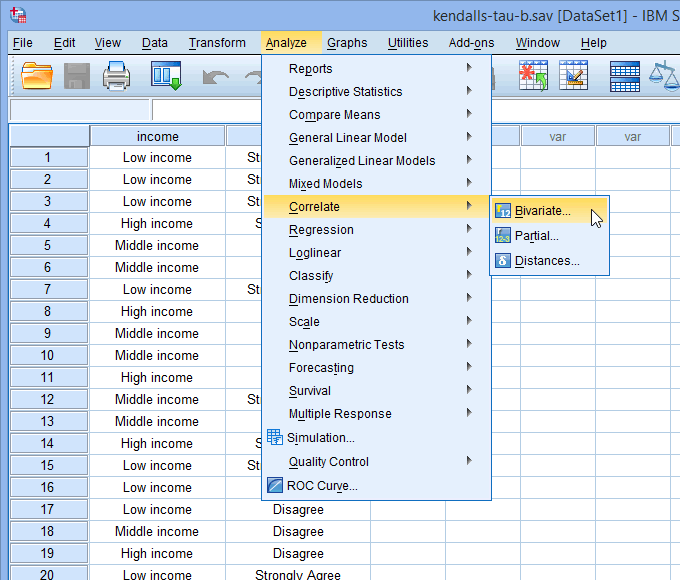Published with written permission from SPSS Statistics, IBM Corporation.

You will be presented with the Bivariate Correlations dialogue box, as shown below: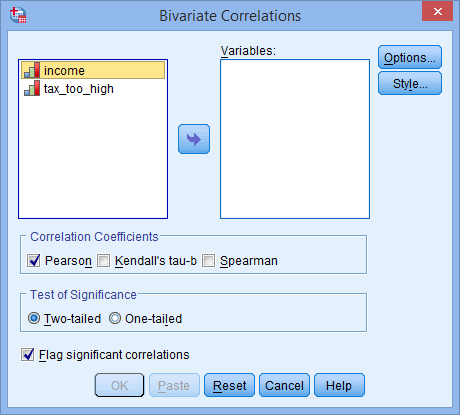Published with written permission from SPSS Statistics, IBM Corporation.

• Transfer the variables income and tax_too_high into the Variables: box by dragging-and-dropping or by clicking the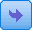button. You will end up with a screen similar to the one below: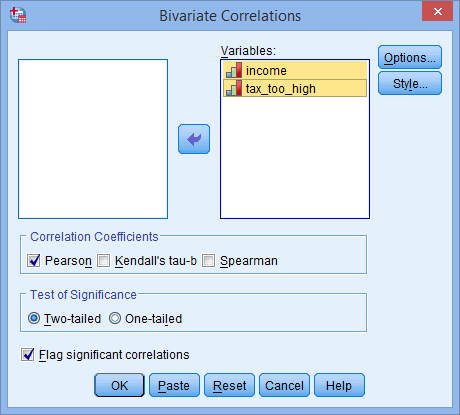Published with written permission from SPSS Statistics, IBM Corporation.

• Make sure that you uncheck the Pearson tick box (it is selected by default in SPSS Statistics) and check the Kendall's tau-b tick box in the –Correlation Coefficients– area, as shown below: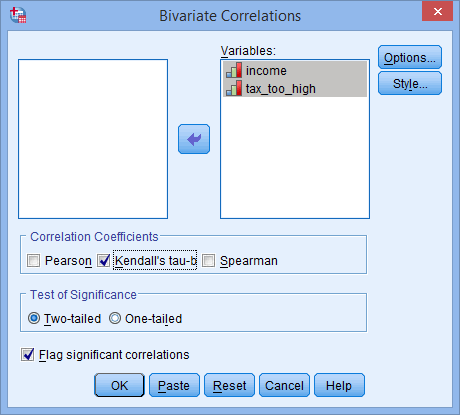Published with written permission from SPSS Statistics, IBM Corporation.

Join the 10,000s of students, academics and professionals who rely on Laerd Statistics.

## Interpreting the Results for Kendall's Tau-b

SPSS Statistics generates one main table for the Kendall's tau-b procedure that you ran in the previous section. If your data passed assumption #2 (i.e., there is a monotonic relationship between your two variables), which we explained earlier in the Assumptions section, you will only need to interpret this one table. However, since you should have tested your data for this assumption, you will also need to interpret the SPSS Statistics output that was produced when you tested for it (i.e., your scatterplot results). Remember that if your data failed this assumption, the output that you get from the Kendall's tau-b procedure (i.e., the table we discuss below) will no longer be correct.

In this "quick start" guide we focus on the results from the Kendall's tau-b procedure only, assuming that your data met this assumption. Therefore, when running the Kendall's tau-b procedure, you will be presented with the table below, entitled Correlations: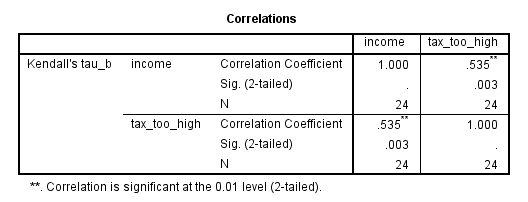Published with written permission from SPSS Statistics, IBM Corporation.

The results are presented in a matrix such that, as can be seen, the correlations are replicated. Nevertheless, the table presents Kendall's tau-b correlation, its significance value and the sample size that the calculation was based on. In this example, we can see that Kendall's tau-b correlation coefficient, τb, is 0.535, and that this is statistically significant (p = 0.003).

## Reporting the Results for Kendall's Tau-b

In our example, you might present the results as follows:

• General

A Kendall's tau-b correlation was run to determine the relationship between income level and views towards income taxes amongst 24 participants. There was a strong, positive correlation between income level and the view that taxes were too high, which was statistically significant (τb = .535, p = .003).

Join the 10,000s of students, academics and professionals who rely on Laerd Statistics.